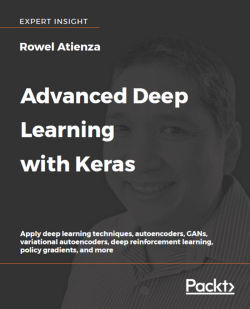# Advanced Deep Learning with Keras4.6 (8 reviews total)
By Rowel Atienza
• \$33.99 eBook
• \$41.99 Print + eBook
What do you get with a Packt Subscription?

• Constantly updated with 100+ new titles each month
• Breadth and depth in over 1,000+ technologies

Recent developments in deep learning, including Generative Adversarial Networks (GANs), Variational Autoencoders (VAEs), and Deep Reinforcement Learning (DRL) are creating impressive AI results in our news headlines - such as AlphaGo Zero beating world chess champions, and generative AI that can create art paintings that sell for over \$400k because they are so human-like.

Advanced Deep Learning with Keras is a comprehensive guide to the advanced deep learning techniques available today, so you can create your own cutting-edge AI. Using Keras as an open-source deep learning library, you'll find hands-on projects throughout that show you how to create more effective AI with the latest techniques.

The journey begins with an overview of MLPs, CNNs, and RNNs, which are the building blocks for the more advanced techniques in the book. You’ll learn how to implement deep learning models with Keras and TensorFlow 1.x, and move forwards to advanced techniques, as you explore deep neural network architectures, including ResNet and DenseNet, and how to create autoencoders. You then learn all about GANs, and how they can open new levels of AI performance. Next, you’ll get up to speed with how VAEs are implemented, and you’ll see how GANs and VAEs have the generative power to synthesize data that can be extremely convincing to humans - a major stride forward for modern AI. To complete this set of advanced techniques, you'll learn how to implement DRL such as Deep Q-Learning and Policy Gradient Methods, which are critical to many modern results in AI.

Publication date:
October 2018
Publisher
Packt
Pages
368
ISBN
9781788629416

## Chapter 1. Introducing Advanced Deep Learning with Keras

In this first chapter, we will introduce the three deep learning artificial neural networks that we will be using throughout the book. These deep learning models are MLPs, CNNs, and RNNs, which are the building blocks to the advanced deep learning topics covered in this book, such as Autoencoders and GANs.

Together, we'll implement these deep learning models using the Keras library in this chapter. We'll start by looking at why Keras is an excellent choice as a tool for us. Next, we'll dig into the installation and implementation details within the three deep learning models.

This chapter will:

• Establish why the Keras library is a great choice to use for advanced deep learning

• Introduce MLPs, CNNs, and RNNs – the core building blocks of most advanced deep learning models, which we'll be using throughout this book

• Provide examples of how to implement MLPs, CNNs, and RNNs using Keras and TensorFlow

• Along the way, start to introduce important deep learning concepts, including optimization, regularization, and loss function

By the end of this chapter, we'll have the fundamental deep learning models implemented using Keras. In the next chapter, we'll get into the advanced deep learning topics that build on these foundations, such as Deep Networks, Autoencoders, and GANs.

## Why is Keras the perfect deep learning library?

Keras [Chollet, François. "Keras (2015)." (2017)] is a popular deep learning library with over 250,000 developers at the time of writing, a number that is more than doubling every year. Over 600 contributors actively maintain it. Some of the examples we'll use in this book have been contributed to the official Keras GitHub repository. Google's TensorFlow, a popular open source deep learning library, uses Keras as a high-level API to its library. In the industry, Keras is used by major technology companies like Google, Netflix, Uber, and NVIDIA. In this chapter, we introduce how to use Keras Sequential API.

We have chosen Keras as our tool of choice to work within this book because Keras is a library dedicated to accelerating the implementation of deep learning models. This makes Keras ideal for when we want to be practical and hands-on, such as when we're exploring the advanced deep learning concepts in this book. Because Keras is intertwined with deep learning, it is essential to learn the key concepts of deep learning before someone can maximize the use of Keras libraries.

### Note

All examples in this book can be found on GitHub at the following link: https://github.com/PacktPublishing/Advanced-Deep-Learning-with-Keras.

Keras is a deep learning library that enables us to build and train models efficiently. In the library, layers are connected to one another like pieces of Lego, resulting in a model that is clean and easy to understand. Model training is straightforward requiring only data, a number of epochs of training, and metrics to monitor. The end result is that most deep learning models can be implemented with a significantly smaller number of lines of code. By using Keras, we'll gain productivity by saving time in code implementation which can instead be spent on more critical tasks such as formulating better deep learning algorithms. We're combining Keras with deep learning, as it offers increased efficiency when introduced with the three deep learning networks that we will introduce in the following sections of this chapter.

Likewise, Keras is ideal for the rapid implementation of deep learning models, like the ones that we will be using in this book. Typical models can be built in few lines of code using the Sequential Model API. However, do not be misled by its simplicity. Keras can also build more advanced and complex models using its API and `Model` and `Layer` classes which can be customized to satisfy unique requirements. Functional API supports building graph-like models, layers reuse, and models that are behaving like Python functions. Meanwhile, `Model` and `Layer` classes provide a framework for implementing uncommon or experimental deep learning models and layers.

### Installing Keras and TensorFlow

Keras is not an independent deep learning library. As shown in Figure 1.1.1, it is built on top of another deep learning library or backend. This could be Google's TensorFlow, MILA's Theano or Microsoft's CNTK. Support for Apache's MXNet is nearly completed. We'll be testing examples in this book on a TensorFlow backend using Python 3. This due to the popularity of TensorFlow, which makes it a common backend.

We can easily switch from one back-end to another by editing the Keras configuration file `.keras/keras.json` in Linux or macOS. Due to the differences in the way low-level algorithms are implemented, networks can often have different speeds on different backends.

On hardware, Keras runs on a CPU, GPU, and Google's TPU. In this book, we'll be testing on a CPU and NVIDIA GPUs (Specifically, the GTX 1060 and GTX 1080Ti models).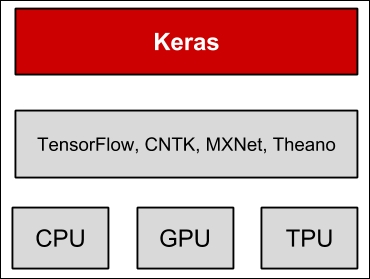Figure 1.1.1: Keras is a high-level library that sits on top of other deep learning models. Keras is supported on CPU, GPU, and TPU.

Before proceeding with the rest of the book, we need to ensure that Keras and TensorFlow are correctly installed. There are multiple ways to perform the installation; one example is installing using `pip3`:

```\$ sudo pip3 install tensorflow
```

If we have a supported NVIDIA GPU, with properly installed drivers, and both NVIDIA's CUDA Toolkit and cuDNN Deep Neural Network library, it is recommended that we install the GPU-enabled version since it can accelerate both training and prediction:

```\$ sudo pip3 install tensorflow-gpu
```

The next step for us is to then install Keras:

```\$ sudo pip3 install keras
```

The examples presented in this book will require additional packages, such as `pydot``pydot_ng`, `vizgraph`, `python3-tk` and `matplotlib`. We'll need to install these packages before proceeding beyond this chapter.

The following should not generate any error if both TensorFlow and Keras are installed along with their dependencies:

```\$ python3
>>> import tensorflow as tf
>>> message = tf.constant('Hello world!')
>>> session = tf.Session()
>>> session.run(message)
b'Hello world!'
>>> import keras.backend as K
Using TensorFlow backend.
>>> print(K.epsilon())
1e-07
```

The warning message about `SSE4.2 AVX AVX2 FMA`, which is similar to the one below can be safely ignored. To remove the warning message, you'll need to recompile and install the TensorFlow source code from https://github.com/tensorflow/tensorflow.

```tensorflow/core/platform/cpu_feature_guard.cc:137] Your CPU supports instructions that this TensorFlow binary was not compiled to use: SSE4.2 AVX AVX2 FMA
```

This book does not cover the complete Keras API. We'll only be covering the materials needed to explain the advanced deep learning topics in this book. For further information, we can consult the official Keras documentation, which can be found at https://keras.io.

## Implementing the core deep learning models - MLPs, CNNs, and RNNs

We've already mentioned that we'll be using three advanced deep learning models, they are:

• MLPs: Multilayer perceptrons

• RNNs: Recurrent neural networks

• CNNs: Convolutional neural networks

These are the three networks that we will be using throughout this book. Despite the three networks being separate, you'll find that they are often combined together in order to take advantage of the strength of each model.

In the following sections of this chapter, we'll discuss these building blocks one by one in more detail. In the following sections, MLPs are covered together with other important topics such as loss function, optimizer, and regularizer. Following on afterward, we'll cover both CNNs and RNNs.

### The difference between MLPs, CNNs, and RNNs

Multilayer perceptrons or MLPs are a fully-connected network. You'll often find them referred to as either deep feedforward networks or feedforward neural networks in some literature. Understanding these networks in terms of known target applications will help us get insights about the underlying reasons for the design of the advanced deep learning models. MLPs are common in simple logistic and linear regression problems. However, MLPs are not optimal for processing sequential and multi-dimensional data patterns. By design, MLPs struggle to remember patterns in sequential data and requires a substantial number of parameters to process multi-dimensional data.

For sequential data input, RNNs are popular because the internal design allows the network to discover dependency in the history of data that is useful for prediction. For multi-dimensional data like images and videos, a CNN excels in extracting feature maps for classification, segmentation, generation, and other purposes. In some cases, a CNN in the form of a 1D convolution is also used for networks with sequential input data. However, in most deep learning models, MLPs, RNNs, and CNNs are combined to make the most out of each network.

MLPs, RNNs, and CNNs do not complete the whole picture of deep networks. There is a need to identify an objective or loss function, an optimizer, and a regularizer. The goal is to reduce the loss function value during training since it is a good guide that a model is learning. To minimize this value, the model employs an optimizer. This is an algorithm that determines how weights and biases should be adjusted at each training step. A trained model must work not only on the training data but also on a test or even on unforeseen input data. The role of the regularizer is to ensure that the trained model generalizes to new data.

## Multilayer perceptrons (MLPs)

The first of the three networks we will be looking at is known as a multilayer perceptrons or (MLPs). Let's suppose that the objective is to create a neural network for identifying numbers based on handwritten digits. For example, when the input to the network is an image of a handwritten number 8, the corresponding prediction must also be the digit 8. This is a classic job of classifier networks that can be trained using logistic regression. To both train and validate a classifier network, there must be a sufficiently large dataset of handwritten digits. The Modified National Institute of Standards and Technology dataset or MNIST for short, is often considered as the Hello World! of deep learning and is a suitable dataset for handwritten digit classification.

Before we discuss the multilayer perceptron model, it's essential that we understand the MNIST dataset. A large number of the examples in this book use the MNIST dataset. MNIST is used to explain and validate deep learning theories because the 70,000 samples it contains are small, yet sufficiently rich in information: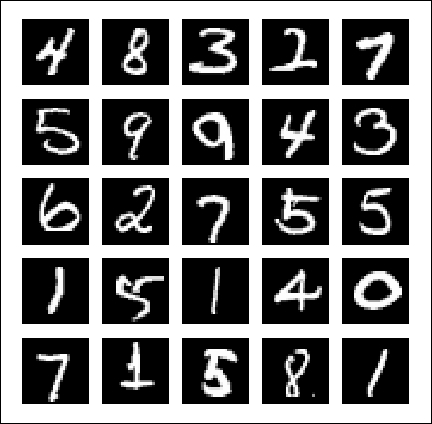Figure 1.3.1: Example images from the MNIST dataset. Each image is 28 × 28-pixel grayscale.

### MNIST dataset

MNIST is a collection of handwritten digits ranging from the number 0 to 9. It has a training set of 60,000 images, and 10,000 test images that are classified into corresponding categories or labels. In some literature, the term target or ground truth is also used to refer to the label.

In the preceding figure sample images of the MNIST digits, each being sized at 28 X 28-pixel grayscale, can be seen. To use the MNIST dataset in Keras, an API is provided to download and extract images and labels automatically. Listing 1.3.1 demonstrates how to load the MNIST dataset in just one line, allowing us to both count the train and test labels and then plot random digit images.

Listing 1.3.1, `mnist-sampler-1.3.1.py`. Keras code showing how to access MNIST dataset, plot 25 random samples, and count the number of labels for train and test datasets:

```import numpy as np
from keras.datasets import mnist
import matplotlib.pyplot as plt

(x_train, y_train), (x_test, y_test) = mnist.load_data()

# count the number of unique train labels
unique, counts = np.unique(y_train, return_counts=True)
print("Train labels: ", dict(zip(unique, counts)))

# count the number of unique test labels
unique, counts = np.unique(y_test, return_counts=True)
print("Test labels: ", dict(zip(unique, counts)))

# sample 25 mnist digits from train dataset
indexes = np.random.randint(0, x_train.shape, size=25)
images = x_train[indexes]
labels = y_train[indexes]

# plot the 25 mnist digits
plt.figure(figsize=(5,5))
for i in range(len(indexes)):
plt.subplot(5, 5, i + 1)
image = images[i]
plt.imshow(image, cmap='gray')
plt.axis('off')

plt.show()
plt.savefig("mnist-samples.png")
plt.close('all')```

The `mnist.load_data()` method is convenient since there is no need to load all 70,000 images and labels individually and store them in arrays. Executing `python3 mnist-sampler-1.3.1.py` on command line prints the distribution of labels in the train and test datasets:

```Train labels:  {0: 5923, 1: 6742, 2: 5958, 3: 6131, 4: 5842, 5: 5421, 6: 5918, 7: 6265, 8: 5851, 9: 5949}
Test labels:  {0: 980, 1: 1135, 2: 1032, 3: 1010, 4: 982, 5: 892, 6: 958, 7: 1028, 8: 974, 9: 1009}
```

Afterward, the code will plot 25 random digits as shown in the preceding figure, Figure 1.3.1.

Before discussing the multilayer perceptron classifier model, it is essential to keep in mind that while MNIST data are 2D tensors, they should be reshaped accordingly depending on the type of input layer. The following figure shows how a 3 × 3 grayscale image is reshaped for MLPs, CNNs, and RNNs input layers: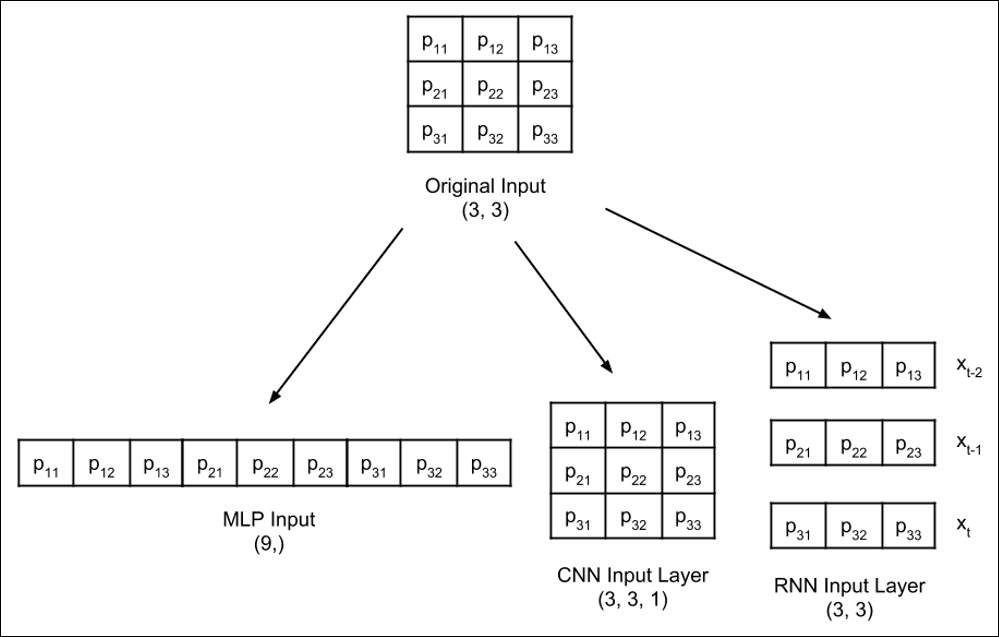Figure 1.3.2: An input image similar to the MNIST data is reshaped depending on the type of input layer. For simplicity, reshaping of a 3 × 3 grayscale image is shown.

### MNIST digits classifier model

The proposed MLP model shown in Figure 1.3.3 can be used for MNIST digit classification. When the units or perceptrons are exposed, the MLP model is a fully connected network as shown in Figure 1.3.4. It will also be shown how the output of the perceptron is computed from inputs as a function of weights, wi and bias, b n for the nth unit. The corresponding Keras implementation is illustrated in Listing 1.3.2.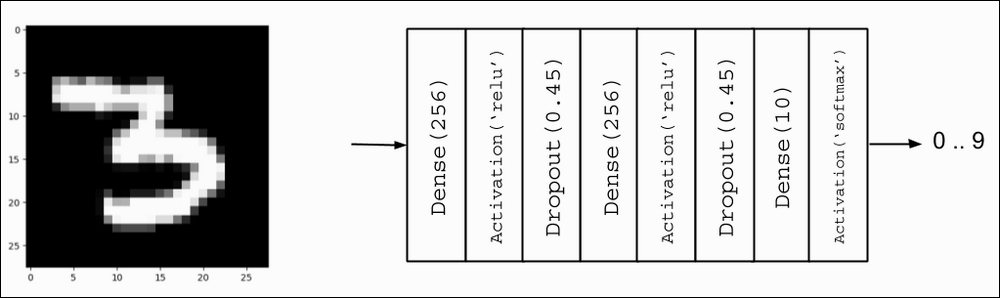Figure 1.3.3: MLP MNIST digit classifier model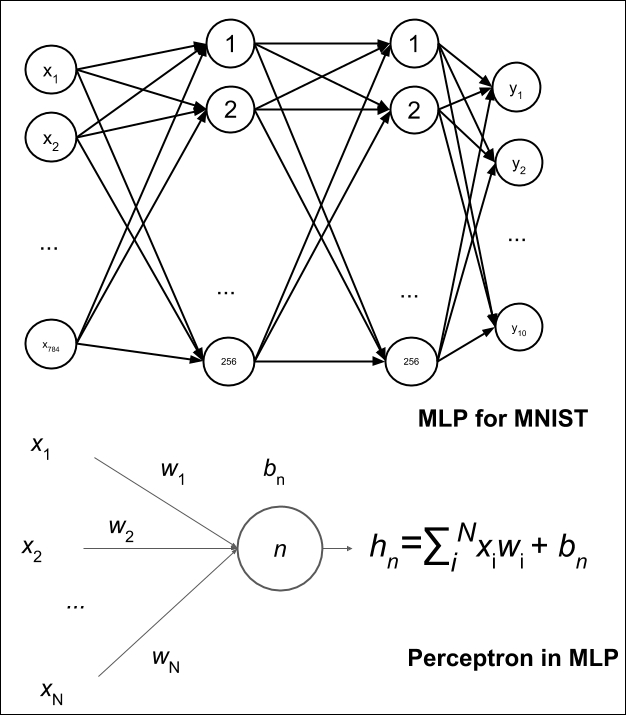Figure 1.3.4: The MLP MNIST digit classifier in Figure 1.3.3 is made up of fully connected layers. For simplicity, the activation and dropout are not shown. One unit or perceptron is also shown.

Listing 1.3.2, `mlp-mnist-1.3.2.py` shows the Keras implementation of the MNIST digit classifier model using MLP:

```import numpy as np
from keras.models import Sequential
from keras.layers import Dense, Activation, Dropout
from keras.utils import to_categorical, plot_model
from keras.datasets import mnist

(x_train, y_train), (x_test, y_test) = mnist.load_data()

# compute the number of labels
num_labels = len(np.unique(y_train))

# convert to one-hot vector
y_train = to_categorical(y_train)
y_test = to_categorical(y_test)

# image dimensions (assumed square)
image_size = x_train.shape
input_size = image_size * image_size

# resize and normalize
x_train = np.reshape(x_train, [-1, input_size])
x_train = x_train.astype('float32') / 255
x_test = np.reshape(x_test, [-1, input_size])
x_test = x_test.astype('float32') / 255

# network parameters
batch_size = 128
hidden_units = 256
dropout = 0.45

# model is a 3-layer MLP with ReLU and dropout after each layer
model = Sequential()
# this is the output for one-hot vector
model.summary()
plot_model(model, to_file='mlp-mnist.png', show_shapes=True)

# loss function for one-hot vector
# accuracy is a good metric for classification tasks
model.compile(loss='categorical_crossentropy',
metrics=['accuracy'])
# train the network
model.fit(x_train, y_train, epochs=20, batch_size=batch_size)

# validate the model on test dataset to determine generalization
loss, acc = model.evaluate(x_test, y_test, batch_size=batch_size)
print("\nTest accuracy: %.1f%%" % (100.0 * acc))```

Before discussing the model implementation, the data must be in the correct shape and format. After loading the MNIST dataset, the number of labels is computed as:

```# compute the number of labels
num_labels = len(np.unique(y_train))```

Hard coding `num_labels = 10` is also an option. But, it's always a good practice to let the computer do its job. The code assumes that `y_train` has labels 0 to 9.

At this point, the labels are in digits format, 0 to 9. This sparse scalar representation of labels is not suitable for the neural network prediction layer that outputs probabilities per class. A more suitable format is called a one-hot vector, a 10-dim vector with all elements 0, except for the index of the digit class. For example, if the label is 2, the equivalent one-hot vector is `[0,0,1,0,0,0,0,0,0,0]`. The first label has index `0`.

The following lines convert each label into a one-hot vector:

```# convert to one-hot vector
y_train = to_categorical(y_train)
y_test = to_categorical(y_test)```

In deep learning, data is stored in tensors. The term tensor applies to a scalar (0D tensor), vector (1D tensor), matrix (2D tensor), and a multi-dimensional tensor. From this point, the term tensor is used unless scalar, vector, or matrix makes the explanation clearer.

The rest computes the image dimensions, `input_size` of the first `Dense` layer and scales each pixel value from 0 to 255 to range from 0.0 to 1.0. Although raw pixel values can be used directly, it is better to normalize the input data as to avoid large gradient values that could make training difficult. The output of the network is also normalized. After training, there is an option to put everything back to the integer pixel values by multiplying the output tensor by 255.

The proposed model is based on MLP layers. Therefore, the input is expected to be a 1D tensor. As such, `x_train` and `x_test` are reshaped to [60000, 28 * 28] and [10000, 28 * 28], respectively.

```# image dimensions (assumed square)
image_size = x_train.shape
input_size = image_size * image_size

# resize and normalize
x_train = np.reshape(x_train, [-1, input_size])
x_train = x_train.astype('float32') / 255
x_test = np.reshape(x_test, [-1, input_size])
x_test = x_test.astype('float32') / 255```

#### Building a model using MLPs and Keras

After data preparation, building the model is next. The proposed model is made of three MLP layers. In Keras, an MLP layer is referred to as Dense, which stands for the densely connected layer. Both the first and second MLP layers are identical in nature with 256 units each, followed by `relu` activation and `dropout`. 256 units are chosen since 128, 512 and 1,024 units have lower performance metrics. At 128 units, the network converges quickly, but has a lower test accuracy. The added number units for 512 or 1,024 does not increase the test accuracy significantly.

The number of units is a hyperparameter. It controls the capacity of the network. The capacity is a measure of the complexity of the function that the network can approximate. For example, for polynomials, the degree is the hyperparameter. As the degree increases, the capacity of the function also increases.

As shown in the following model, the classifier model is implemented using a sequential model API of Keras. This is sufficient if the model requires one input and one output processed by a sequence of layers. For simplicity, we'll use this in the meantime, however, in Chapter 2, Deep Neural Networks, the Functional API of Keras will be introduced to implement advanced deep learning models.

```# model is a 3-layer MLP with ReLU and dropout after each layer
model = Sequential()
# this is the output for one-hot vector

Since a `Dense` layer is a linear operation, a sequence of `Dense` layers can only approximate a linear function. The problem is that the MNIST digit classification is inherently a non-linear process. Inserting a `relu` activation between `Dense` layers will enable MLPs to model non-linear mappings. `relu` or Rectified Linear Unit (ReLU) is a simple non-linear function. It's very much like a filter that allows positive inputs to pass through unchanged while clamping everything else to zero. Mathematically, `relu` is expressed in the following equation and plotted in Figure 1.3.5:

relu(x) = max(0,x)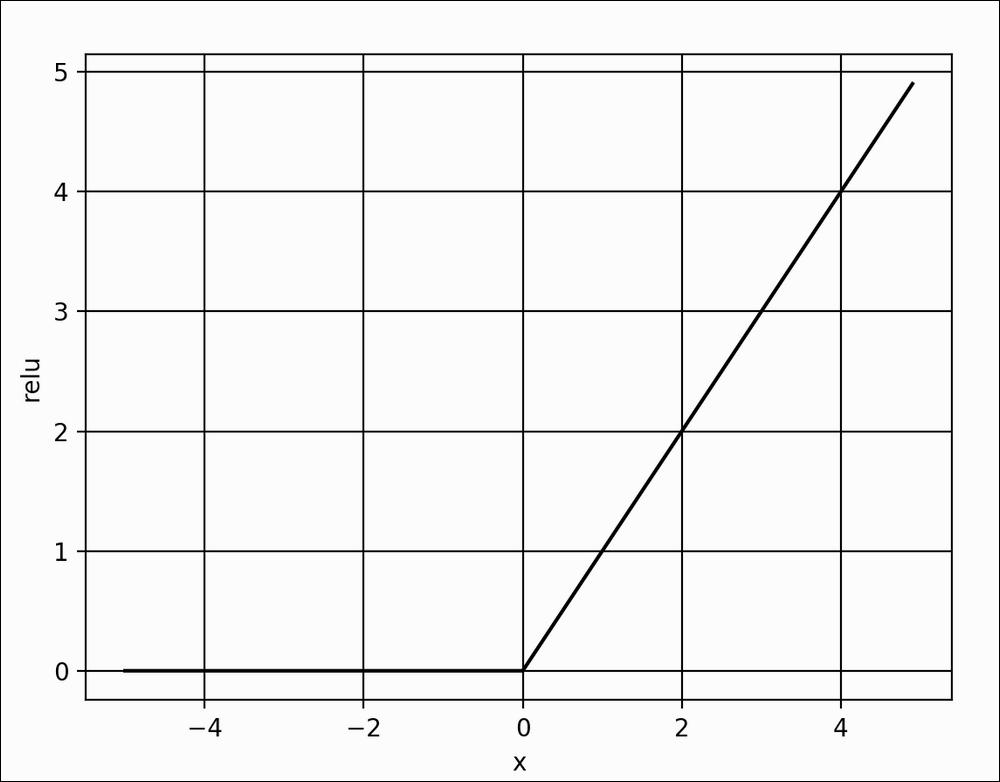Figure 1.3.5: Plot of ReLU function. The ReLU function introduces non-linearity in neural networks.

There are other non-linear functions that can be used such as `elu`, `selu`, `softplus`, `sigmoid`, and `tanh`. However, `relu` is the most commonly used in the industry and is computationally efficient due to its simplicity. The `sigmoid` and `tanh` are used as activation functions in the output layer and described later. Table 1.3.1 shows the equation for each of these activation functions:

 `relu` relu(x) = max(0,x) 1.3.1 `softplus` softplus(x) = log(1 + e x) 1.3.2 `elu`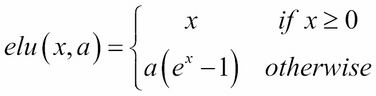where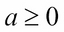and is a tunable hyperparameter 1.3.3 `selu` selu(x) = k × elu(x,a) where k = 1.0507009873554804934193349852946 and a = 1.6732632423543772848170429916717 1.3.4

Table 1.3.1: Definition of common non-linear activation functions

### Regularization

A neural network has the tendency to memorize its training data especially if it contains more than enough capacity. In such a case, the network fails catastrophically when subjected to the test data. This is the classic case of the network failing to generalize. To avoid this tendency, the model uses a regularizing layer or function. A common regularizing layer is referred to as a dropout.

The idea of dropout is simple. Given a dropout rate (here, it is set to `dropout=0.45`), the `Dropout` layer randomly removes that fraction of units from participating in the next layer. For example, if the first layer has 256 units, after `dropout=0.45 `is applied, only (1 - 0.45) * 256 units = 140 units from layer 1 participate in layer 2. The `Dropout` layer makes neural networks robust to unforeseen input data because the network is trained to predict correctly, even if some units are missing. It's worth noting that dropout is not used in the output layer and it is only active during training. Moreover, dropout is not present during prediction.

There are regularizers that can be used other than dropouts like `l1` or `l2`. In Keras, the bias, weight and activation output can be regularized per layer. `l1` and `l2` favor smaller parameter values by adding a penalty function. Both `l1` and `l2` enforce the penalty using a fraction of the sum of absolute (`l1`) or square (`l2`) of parameter values. In other words, the penalty function forces the optimizer to find parameter values that are small. Neural networks with small parameter values are more insensitive to the presence of noise from within the input data.

As an example, `l2` weight regularizer with `fraction=0.001` can be implemented as:

```from keras.regularizers import l2
kernel_regularizer=l2(0.001),
input_dim=input_size))```

No additional layer is added if `l1` or `l2` regularization is used. The regularization is imposed in the `Dense` layer internally. For the proposed model, dropout still has a better performance than `l2`.

### Output activation and loss function

The output layer has 10 units followed by `softmax` activation. The 10 units correspond to the 10 possible labels, classes or categories. The `softmax` activation can be expressed mathematically as shown in the following equation: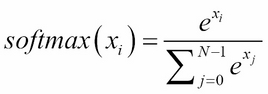(Equation 1.3.5)

The equation is applied to all N = 10 outputs, x i for i = 0, 1 … 9 for the final prediction. The idea of `softmax` is surprisingly simple. It squashes the outputs into probabilities by normalizing the prediction. Here, each predicted output is a probability that the index is the correct label of the given input image. The sum of all the probabilities for all outputs is 1.0. For example, when the `softmax` layer generates a prediction, it will be a 10-dim 1D tensor that may look like the following output:

```[  3.57351579e-11   7.08998016e-08   2.30154569e-07   6.35787558e-07
5.57471187e-11   4.15353840e-09   3.55973775e-16   9.99995947e-01
1.29531730e-09   3.06023480e-06]
```

The prediction output tensor suggests that the input image is going to be 7 given that its index has the highest probability. The `numpy.argmax()` method can be used to determine the index of the element with the highest value.

There are other choices of output activation layer, like `linear`, `sigmoid`, and `tanh`. The `linear` activation is an identity function. It copies its input to its output. The `sigmoid` function is more specifically known as a logistic sigmoid. This will be used if the elements of the prediction tensor should be mapped between 0.0 and 1.0 independently. The summation of all elements of the predicted tensor is not constrained to 1.0 unlike in `softmax`. For example, `sigmoid` is used as the last layer in sentiment prediction (0.0 is bad to 1.0, which is good) or in image generation (0.0 is 0 to 1.0 is 255-pixel values).

The `tanh` function maps its input in the range -1.0 to 1.0. This is important if the output can swing in both positive and negative values. The `tanh` function is more popularly used in the internal layer of recurrent neural networks but has also been used as output layer activation. If `tanh` is used to replace `sigmoid` in the output activation, the data used must be scaled appropriately. For example, instead of scaling each grayscale pixel in the range [0.0 1.0] using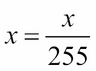, it is assigned in the range [-1.0 1.0] by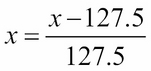.

The following graph shows the `sigmoid` and `tanh` functions. Mathematically, `sigmoid` can be expressed in equation as follows: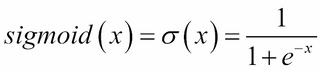(Equation 1.3.6)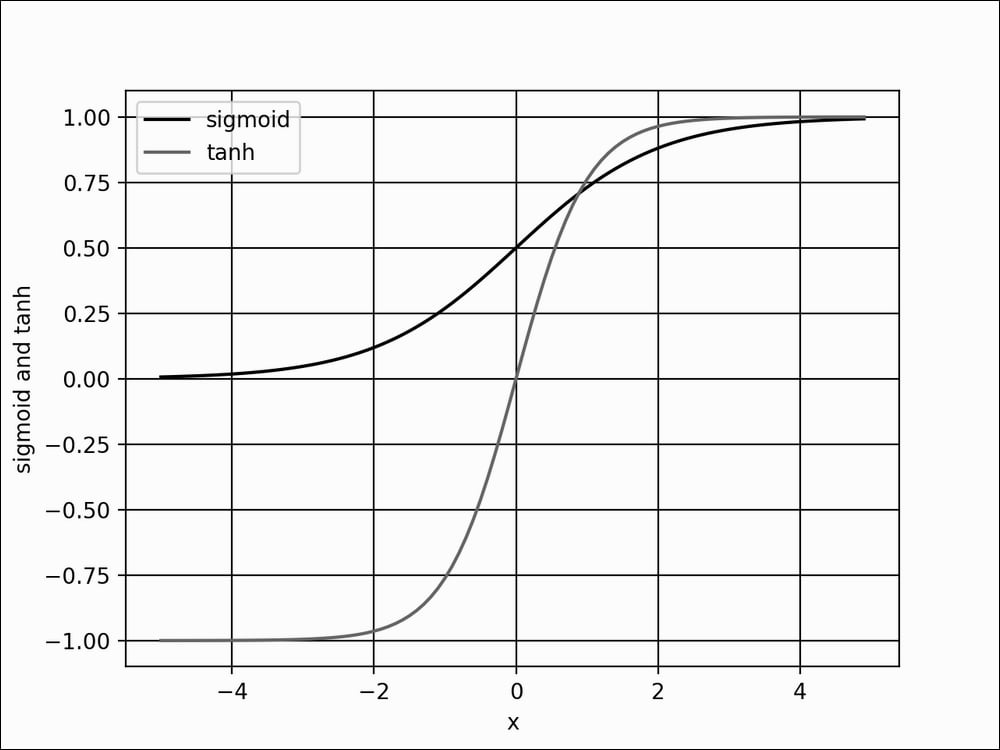Figure 1.3.6: Plots of sigmoid and tanh

How far the predicted tensor is from the one-hot ground truth vector is called loss. One type of loss function is `mean_squared_error` (mse), or the average of the squares of the differences between target and prediction. In the current example, we are using `categorical_crossentropy`. It's the negative of the sum of the product of the target and the logarithm of the prediction. There are other loss functions that are available in Keras, such as `mean_absolute_error`, and `binary_crossentropy`. The choice of the loss function is not arbitrary but should be a criterion that the model is learning. For classification by category, `categorical_crossentropy` or `mean_squared_error` is a good choice after the `softmax` activation layer. The `binary_crossentropy` loss function is normally used after the `sigmoid` activation layer while `mean_squared_error` is an option for `tanh` output.

### Optimization

With optimization, the objective is to minimize the loss function. The idea is that if the loss is reduced to an acceptable level, the model has indirectly learned the function mapping input to output. Performance metrics are used to determine if a model has learned the underlying data distribution. The default metric in Keras is loss. During training, validation, and testing, other metrics such as accuracy can also be included. Accuracy is the percent, or fraction, of correct predictions based on ground truth. In deep learning, there are many other performance metrics. However, it depends on the target application of the model. In literature, performance metrics of the trained model on the test dataset is reported for comparison to other deep learning models.

In Keras, there are several choices for optimizers. The most commonly used optimizers are; Stochastic Gradient Descent (SGD), Adaptive Moments (Adam), and Root Mean Squared Propagation (RMSprop). Each optimizer features tunable parameters like learning rate, momentum, and decay. Adam and RMSprop are variations of SGD with adaptive learning rates. In the proposed classifier network, Adam is used since it has the highest test accuracy.

SGD is considered the most fundamental optimizer. It's a simpler version of the gradient descent in calculus. In gradient descent (GD), tracing the curve of a function downhill finds the minimum value, much like walking downhill in a valley or opposite the gradient until the bottom is reached.

The GD algorithm is illustrated in Figure 1.3.7. Let's suppose x is the parameter (for example, weight) being tuned to find the minimum value of y (for example, loss function). Starting at an arbitrary point of x = -0.5 with the gradient being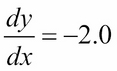. The GD algorithm imposes that x is then updated to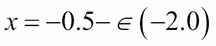. The new value of x is equal to the old value, plus the opposite of the gradient scaled by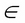. The small numberrefers to the learning rate. If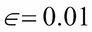, then the new value of x = -0.48.

GD is performed iteratively. At each step, y will get closer to its minimum value. At x = 0.5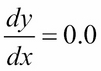, the GD has found the absolute minimum value of y = -1.25. The gradient recommends no further change in x.

The choice of learning rate is crucial. A large value ofmay not find the minimum value since the search will just swing back and forth around the minimum value. On the other hand, too small value ofmay take a significant number of iterations before the minimum is found. In the case of multiple minima, the search might get stuck in a local minimum.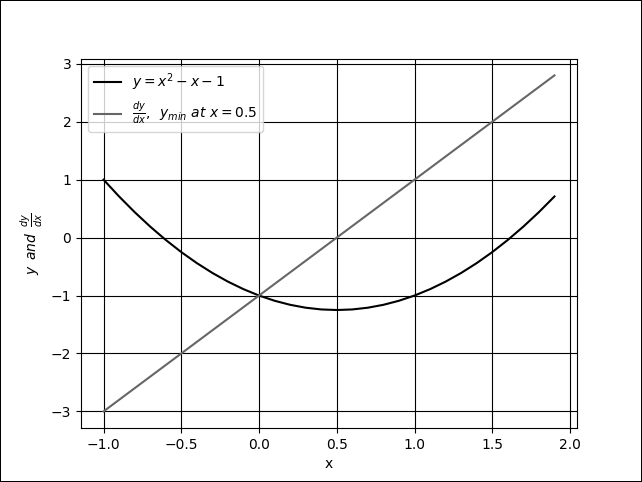Figure 1.3.7: Gradient descent is similar to walking downhill on the function curve until the lowest point is reached. In this plot, the global minimum is at x = 0.5.

An example of multiple minima can be seen in Figure 1.3.8. If for some reason the search started at the left side of the plot and the learning rate is very small, there is a high probability that GD will find x = -1.51 as the minimum value of y. GD will not find the global minimum at x = 1.66. A sufficiently valued learning rate will enable the gradient descent to overcome the hill at x = 0.0. In deep learning practice, it is normally recommended to start at a bigger learning rate (for example. 0.1 to 0.001) and gradually decrease as the loss gets closer to the minimum.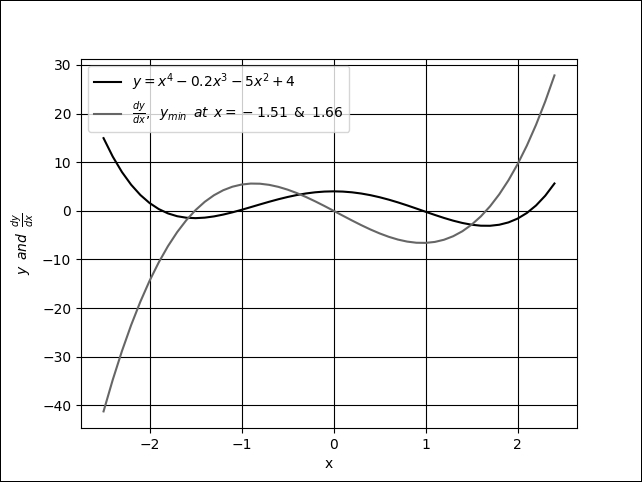Figure 1.3.8: Plot of a function with 2 minima, x = -1.51 and x = 1.66. Also shown is the derivative of the function.

Gradient descent is not typically used in deep neural networks since you'll often come upon millions of parameters that need to be trained. It is computationally inefficient to perform a full gradient descent. Instead, SGD is used. In SGD, a mini batch of samples is chosen to compute an approximate value of the descent. The parameters (for example, weights and biases) are adjusted by the following equation: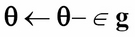(Equation 1.3.7)

In this equation,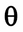and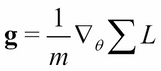are the parameters and gradients tensor of the loss function respectively. The g is computed from partial derivatives of the loss function. The mini-batch size is recommended to be a power of 2 for GPU optimization purposes. In the proposed network, `batch_size=128`.

Equation 1.3.7 computes the last layer parameter updates. So, how do we adjust the parameters of the preceding layers? For this case, the chain rule of differentiation is applied to propagate the derivatives to the lower layers and compute the gradients accordingly. This algorithm is known as backpropagation in deep learning. The details of backpropagation are beyond the scope of this book. However, a good online reference can be found at http://neuralnetworksanddeeplearning.com.

Since optimization is based on differentiation, it follows that an important criterion of the loss function is that it must be smooth or differentiable. This is an important constraint to keep in mind when introducing a new loss function.

Given the training dataset, the choice of the loss function, the optimizer, and the regularizer, the model can now be trained by calling the `fit()` function:

```# loss function for one-hot vector
# accuracy is a good metric for classification tasks
model.compile(loss='categorical_crossentropy',
metrics=['accuracy'])
# train the network
model.fit(x_train, y_train, epochs=20, batch_size=batch_size)```

This is another helpful feature of Keras. By just supplying both the x and y data, the number of epochs to train, and the batch size, `fit()` does the rest. In other deep learning frameworks, this translates to multiple tasks such as preparing the input and output data in the proper format, loading, monitoring, and so on. While all of these must be done inside a `for` loop! In Keras, everything is done in just one line.

In the `fit()` function, an epoch is the complete sampling of the entire training data. The `batch_size` parameter is the sample size of the number of inputs to process at each training step. To complete one epoch, `fit()` requires the size of train dataset divided by batch size, plus 1 to compensate for any fractional part.

### Performance evaluation

At this point, the model for the MNIST digit classifier is now complete. Performance evaluation will be the next crucial step to determine if the proposed model has come up with a satisfactory solution. Training the model for 20 epochs will be sufficient to obtain comparable performance metrics.

The following table, Table 1.3.2, shows the different network configurations and corresponding performance measures. Under Layers, the number of units is shown for layers 1 to 3. For each optimizer, the default parameters in Keras are used. The effects of varying the regularizer, optimizer and number of units per layer can be observed. Another important observation in Table 1.3.2 is that bigger networks do not necessarily translate to better performance.

Increasing the depth of this network shows no added benefits in terms of accuracy for both training and testing datasets. On the other hand, a smaller number of units, like 128, could also lower both the test and train accuracy. The best train accuracy at 99.93% is obtained when the regularizer is removed, and 256 units per layer are used. The test accuracy, however, is much lower at 98.0%, as a result of the network overfitting.

The highest test accuracy is with the Adam optimizer and `Dropout(0.45)` at 98.5%. Technically, there is still some degree of overfitting given that its training accuracy is 99.39%. Both the train and test accuracy are the same at 98.2% for 256-512-256, `Dropout(0.45)` and SGD. Removing both the Regularizer and ReLU layers results in it having the worst performance. Generally, we'll find that the `Dropout` layer has better performance than `l2`.

Following table demonstrates a typical deep neural network performance during tuning. The example indicates that there is a need to improve the network architecture. In the following section, another model using CNNs shows a significant improvement in test accuracy:

Layers

Regularizer

Optimizer

ReLU

Train Accuracy, %

Test Accuracy, %

256-256-256

None

SGD

None

93.65

92.5

256-256-256

L2(0.001)

SGD

Yes

99.35

98.0

256-256-256

L2(0.01)

SGD

Yes

96.90

96.7

256-256-256

None

SGD

Yes

99.93

98.0

256-256-256

Dropout(0.4)

SGD

Yes

98.23

98.1

256-256-256

Dropout(0.45)

SGD

Yes

98.07

98.1

256-256-256

Dropout(0.5)

SGD

Yes

97.68

98.1

256-256-256

Dropout(0.6)

SGD

Yes

97.11

97.9

256-512-256

Dropout(0.45)

SGD

Yes

98.21

98.2

512-512-512

Dropout(0.2)

SGD

Yes

99.45

98.3

512-512-512

Dropout(0.4)

SGD

Yes

98.95

98.3

512-1024-512

Dropout(0.45)

SGD

Yes

98.90

98.2

1024-1024-1024

Dropout(0.4)

SGD

Yes

99.37

98.3

256-256-256

Dropout(0.6)

Yes

98.64

98.2

256-256-256

Dropout(0.55)

Yes

99.02

98.3

256-256-256

Dropout(0.45)

Yes

99.39

98.5

256-256-256

Dropout(0.45)

RMSprop

Yes

98.75

98.1

128-128-128

Dropout(0.45)

Yes

98.70

97.7

### Model summary

Using the Keras library provides us with a quick mechanism to double check the model description by calling:

`model.summary()`

Listing 1.3.2 shows the model summary of the proposed network. It requires a total of 269,322 parameters. This is substantial considering that we have a simple task of classifying MNIST digits. MLPs are not parameter efficient. The number of parameters can be computed from Figure 1.3.4 by focusing on how the output of the perceptron is computed. From input to Dense layer: 784 × 256 + 256 = 200,960. From first Dense to second Dense: 256 × 256 + 256 = 65,792. From second Dense to the output layer: 10 × 256 + 10 = 2,570. The total is 269,322.

Listing 1.3.2 shows a summary of an MLP MNIST digit classifier model:

```_________________________________________________________________
Layer (type)                 Output Shape              Param #
=================================================================
dense_1 (Dense)              (None, 256)               200960
_________________________________________________________________
activation_1 (Activation)    (None, 256)               0
_________________________________________________________________
dropout_1 (Dropout)          (None, 256)               0
_________________________________________________________________
dense_2 (Dense)              (None, 256)               65792
_________________________________________________________________
activation_2 (Activation)    (None, 256)               0
_________________________________________________________________
dropout_2 (Dropout)          (None, 256)               0
_________________________________________________________________
dense_3 (Dense)              (None, 10)                2570
_________________________________________________________________
activation_3 (Activation)    (None, 10)                0
=================================================================
Total params: 269,322
Trainable params: 269,322
Non-trainable params: 0
```

Another way of verifying the network is by calling:

```plot_model(model, to_file='mlp-mnist.png', show_shapes=True)
```

Figure 1.3.9 shows the plot. You'll find that this is similar to the results of `summary()` but graphically shows the interconnection and I/O of each layer.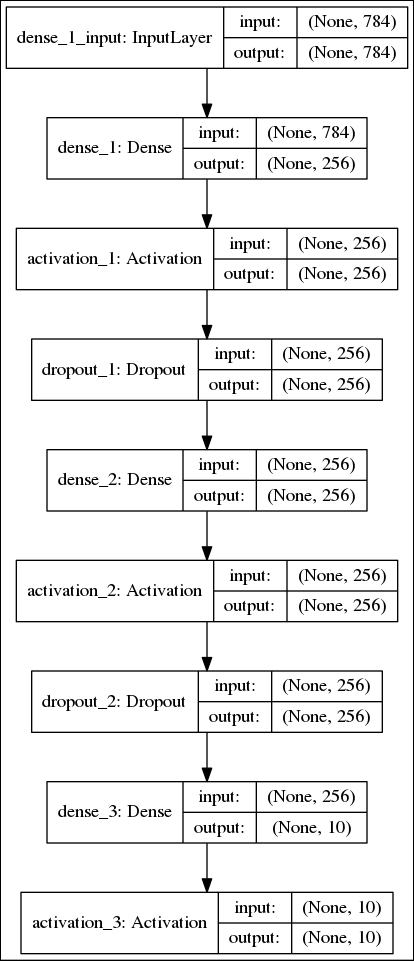Figure 1.3.9: The graphical description of the MLP MNIST digit classifier

## Convolutional neural networks (CNNs)

We're now going to move onto the second artificial neural network, Convolutional Neural Networks (CNNs). In this section, we're going solve the same MNIST digit classification problem, instead this time using CNNs.

Figure 1.4.1 shows the CNN model that we'll use for the MNIST digit classification, while its implementation is illustrated in Listing 1.4.1. Some changes in the previous model will be needed to implement the CNN model. Instead of having input vector, the input tensor now has new dimensions (height, width, channels) or (image_size, image_size, 1) = (28, 28, 1) for the grayscale MNIST images. Resizing the train and test images will be needed to conform to this input shape requirement.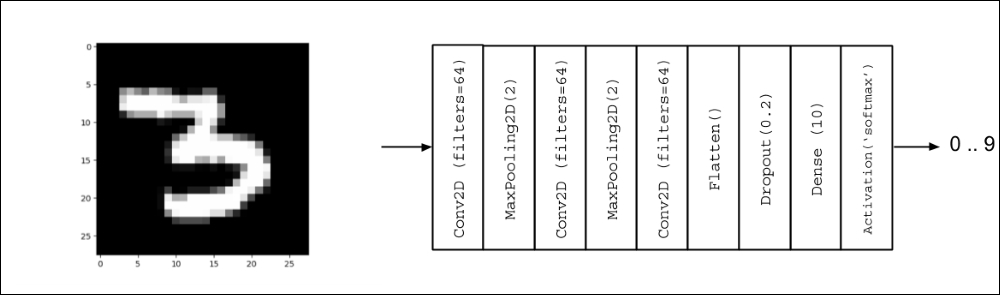Figure 1.4.1: CNN model for MNIST digit classification

Listing 1.4.1, `cnn-mnist-1.4.1.py` shows the Keras code for the MNIST digit classification using CNN:

```import numpy as np
from keras.models import Sequential
from keras.layers import Activation, Dense, Dropout
from keras.layers import Conv2D, MaxPooling2D, Flatten
from keras.utils import to_categorical, plot_model
from keras.datasets import mnist

(x_train, y_train), (x_test, y_test) = mnist.load_data()

# compute the number of labels
num_labels = len(np.unique(y_train))

# convert to one-hot vector
y_train = to_categorical(y_train)
y_test = to_categorical(y_test)

# input image dimensions
image_size = x_train.shape
# resize and normalize
x_train = np.reshape(x_train,[-1, image_size, image_size, 1])
x_test = np.reshape(x_test,[-1, image_size, image_size, 1])
x_train = x_train.astype('float32') / 255
x_test = x_test.astype('float32') / 255

# network parameters
# image is processed as is (square grayscale)
input_shape = (image_size, image_size, 1)
batch_size = 128
kernel_size = 3
pool_size = 2
filters = 64
dropout = 0.2

# model is a stack of CNN-ReLU-MaxPooling
model = Sequential()
kernel_size=kernel_size,
activation='relu',
input_shape=input_shape))
kernel_size=kernel_size,
activation='relu'))
kernel_size=kernel_size,
activation='relu'))
# output layer is 10-dim one-hot vector
model.summary()
plot_model(model, to_file='cnn-mnist.png', show_shapes=True)

# loss function for one-hot vector
# accuracy is good metric for classification tasks
model.compile(loss='categorical_crossentropy',
metrics=['accuracy'])
# train the network
model.fit(x_train, y_train, epochs=10, batch_size=batch_size)

loss, acc = model.evaluate(x_test, y_test, batch_size=batch_size)
print("\nTest accuracy: %.1f%%" % (100.0 * acc))```

The major change here is the use of `Conv2D` layers. The `relu` activation function is already an argument of `Conv2D`. The `relu` function can be brought out as an `Activation` layer when the batch normalization layer is included in the model. Batch normalization is used in deep CNNs so that large learning rates can be used without causing instability during training.

### Convolution

If in the MLP model the number of units characterizes the `Dense` layers, the kernel characterizes the CNN operations. As shown in Figure 1.4.2, the kernel can be visualized as a rectangular patch or window that slides through the whole image from left to right, and top to bottom. This operation is called convolution. It transforms the input image into a feature maps, which is a representation of what the kernel has learned from the input image. The feature maps are then transformed into another feature maps in the succeeding layer and so on. The number of feature maps generated per `Conv2D` is controlled by the `filters` argument.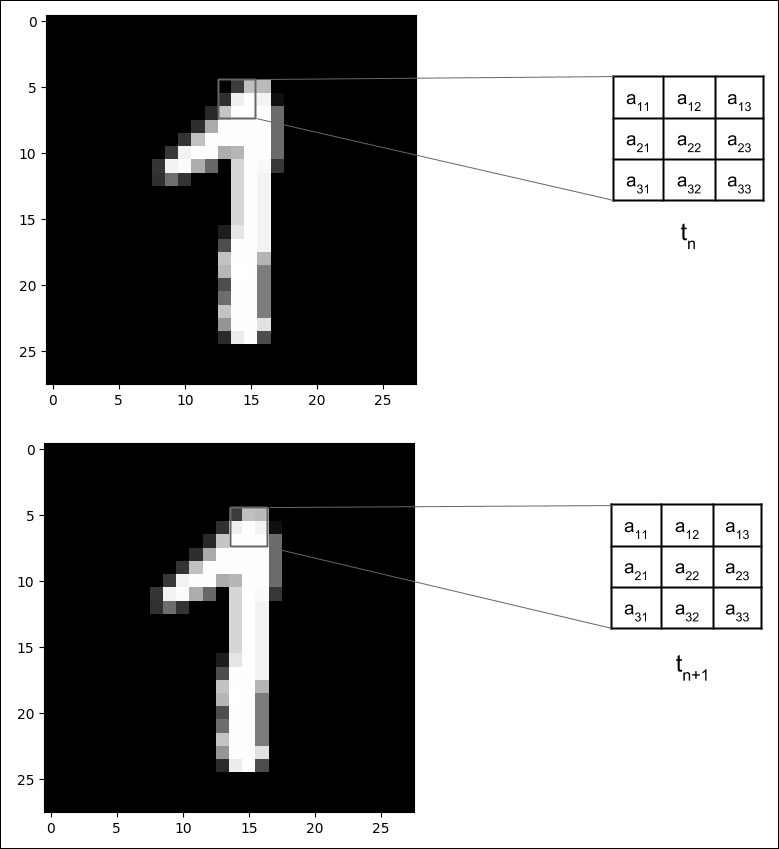Figure 1.4.2: A 3 × 3 kernel is convolved with an MNIST digit image. The convolution is shown in steps tn and tn+1 where the kernel moved by a stride of 1 pixel to the right.

The computation involved in the convolution is shown in Figure 1.4.3. For simplicity, a 5 × 5 input image (or input feature map) where a 3 × 3 kernel is applied is illustrated. The resulting feature map is shown after the convolution. The value of one element of the feature map is shaded. You'll notice that the resulting feature map is smaller than the original input image, this is because the convolution is only performed on valid elements. The kernel cannot go beyond the borders of the image. If the dimensions of the input should be the same as the output feature maps, `Conv2D` will accept the option `padding='same'`. The input is padded with zeroes around its borders to keep the dimensions unchanged after the convolution: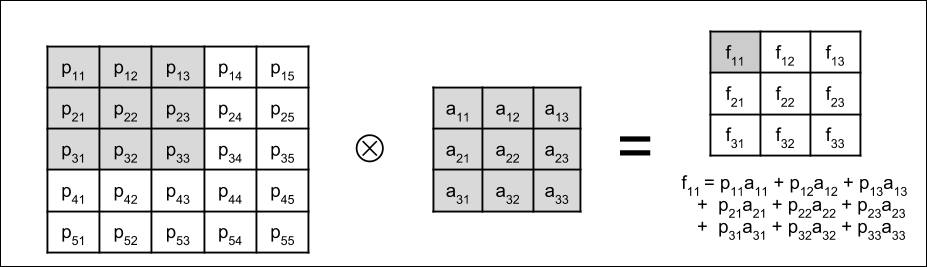Figure 1.4.3: The convolution operation shows how one element of the feature map is computed

### Pooling operations

The last change is the addition of a `MaxPooling2D` layer with the argument `pool_size=2`. `MaxPooling2D `compresses each feature map. Every patch of size `pool_size` × `pool_size` is reduced to one pixel. The value is equal to the maximum pixel value within the patch. `MaxPooling2D `is shown in the following figure for two patches: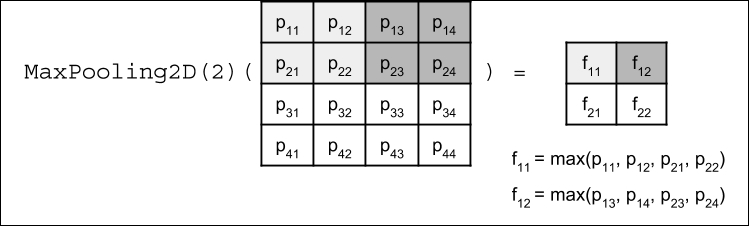Figure 1.4.4: MaxPooling2D operation. For simplicity, the input feature map is 4 × 4 resulting in a 2 × 2 feature map.

The significance of `MaxPooling2D` is the reduction in feature maps size which translates to increased kernel coverage. For example, after `MaxPooling2D(2)`, the 2 × 2 kernel is now approximately convolving with a 4 × 4 patch. The CNN has learned a new set of feature maps for a different coverage.

There are other means of pooling and compression. For example, to achieve a 50% size reduction as `MaxPooling2D(2)`, `AveragePooling2D(2)` takes the average of a patch instead of finding the maximum. Strided convolution, `Conv2D(strides=2,…)` will skip every two pixels during convolution and will still have the same 50% size reduction effect. There are subtle differences in the effectiveness of each reduction technique.

In `Conv2D` and `MaxPooling2D`, both `pool_size` and `kernel` can be non-square. In these cases, both the row and column sizes must be indicated. For example, `pool_size=(1, 2)` and `kernel=(3, 5)`.

The output of the last `MaxPooling2D` is a stack of feature maps. The role of `Flatten` is to convert the stack of feature maps into a vector format that is suitable for either `Dropout` or `Dense` layers, similar to the MLP model output layer.

### Performance evaluation and model summary

As shown in Listing 1.4.2, the CNN model in Listing 1.4.1 requires a smaller number of parameters at 80,226 compared to 269,322 when MLP layers are used. The `conv2d_1` layer has 640 parameters because each kernel has 3 × 3 = 9 parameters, and each of the 64 feature maps has one kernel and one bias parameter. The number of parameters for other convolution layers can be computed in a similar way. Figure 1.4.5 shows the graphical representation of the CNN MNIST digit classifier.

Table 1.4.1 shows that the maximum test accuracy of 99.4% which can be achieved for a 3–layer network with 64 feature maps per layer using the Adam optimizer with `dropout=0.2`. CNNs are more parameter efficient and have a higher accuracy than MLPs. Likewise, CNNs are also suitable for learning representations from sequential data, images, and videos.

Listing 1.4.2 shows a summary of a CNN MNIST digit classifier:

```_________________________________________________________________
Layer (type)                 Output Shape              Param #
=================================================================
conv2d_1 (Conv2D)            (None, 26, 26, 64)        640
_________________________________________________________________
max_pooling2d_1 (MaxPooling2 (None, 13, 13, 64)        0
_________________________________________________________________
conv2d_2 (Conv2D)            (None, 11, 11, 64)        36928
_________________________________________________________________
max_pooling2d_2 (MaxPooling2 (None, 5, 5, 64)          0
_________________________________________________________________
conv2d_3 (Conv2D)            (None, 3, 3, 64)          36928
_________________________________________________________________
flatten_1 (Flatten)          (None, 576)               0
_________________________________________________________________
dropout_1 (Dropout)          (None, 576)               0
_________________________________________________________________
dense_1 (Dense)              (None, 10)                5770
_________________________________________________________________
activation_1 (Activation)    (None, 10)                0
=================================================================
Total params: 80,266
Trainable params: 80,266
Non-trainable params: 0
```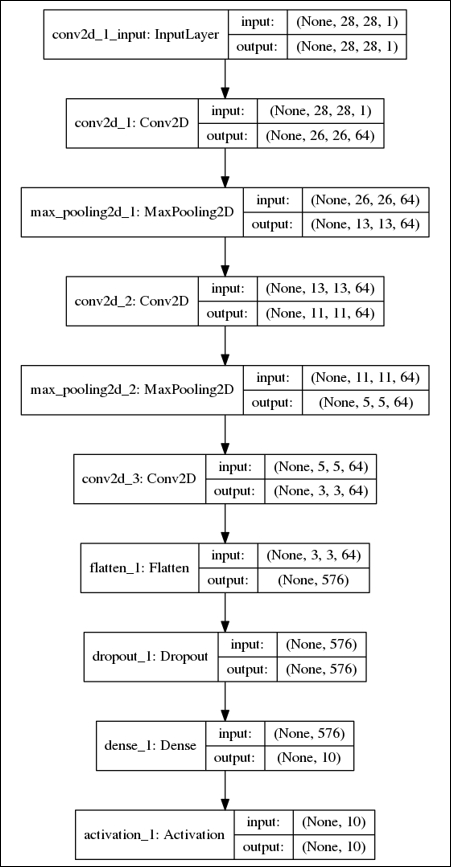Figure 1.4.5: Graphical description of the CNN MNIST digit classifier

Layers

Optimizer

Regularizer

Train Accuracy, %

Test Accuracy, %

64-64-64

SGD

Dropout(0.2)

97.76

98.50

64-64-64

RMSprop

Dropout(0.2)

99.11

99.00

64-64-64

Dropout(0.2)

99.75

99.40

64-64-64

Dropout(0.4)

99.64

99.30

## Recurrent neural networks (RNNs)

We're now going to look at the last of our three artificial neural networks, Recurrent neural networks, or RNNs.

RNNs are a family of networks that are suitable for learning representations of sequential data like text in Natural Language Processing (NLP) or stream of sensor data in instrumentation. While each MNIST data sample is not sequential in nature, it is not hard to imagine that every image can be interpreted as a sequence of rows or columns of pixels. Thus, a model based on RNNs can process each MNIST image as a sequence of 28-element input vectors with timesteps equal to 28. The following listing shows the code for the RNN model in Figure 1.5.1: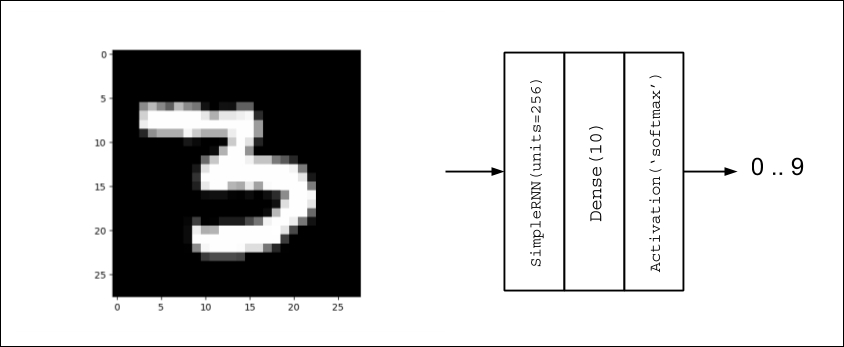Figure 1.5.1: RNN model for MNIST digit classification

In the following listing, Listing 1.5.1, the `rnn-mnist-1.5.1.py` shows the Keras code for MNIST digit classification using RNNs:

```import numpy as np
from keras.models import Sequential
from keras.layers import Dense, Activation, SimpleRNN
from keras.utils import to_categorical, plot_model
from keras.datasets import mnist

(x_train, y_train), (x_test, y_test) = mnist.load_data()

# compute the number of labels
num_labels = len(np.unique(y_train))

# convert to one-hot vector
y_train = to_categorical(y_train)
y_test = to_categorical(y_test)

# resize and normalize
image_size = x_train.shape
x_train = np.reshape(x_train,[-1, image_size, image_size])
x_test = np.reshape(x_test,[-1, image_size, image_size])
x_train = x_train.astype('float32') / 255
x_test = x_test.astype('float32') / 255

# network parameters
input_shape = (image_size, image_size)
batch_size = 128
units = 256
dropout = 0.2

# model is RNN with 256 units, input is 28-dim vector 28 timesteps
model = Sequential()
dropout=dropout,
input_shape=input_shape))
model.summary()
plot_model(model, to_file='rnn-mnist.png', show_shapes=True)

# loss function for one-hot vector
# use of sgd optimizer
# accuracy is good metric for classification tasks
model.compile(loss='categorical_crossentropy',
optimizer='sgd',
metrics=['accuracy'])
# train the network
model.fit(x_train, y_train, epochs=20, batch_size=batch_size)

loss, acc = model.evaluate(x_test, y_test, batch_size=batch_size)
print("\nTest accuracy: %.1f%%" % (100.0 * acc))```

There are the two main differences between RNNs and the two previous models. First is the `input_shape = (image_size, image_size)` which is actually `input_shape = (timesteps, input_dim)` or a sequence of `input_dim`—dimension vectors of `timesteps` length. Second is the use of a `SimpleRNN` layer to represent an RNN cell with `units=256`. The `units` variable represents the number of output units. If the CNN is characterized by the convolution of kernel across the input feature map, the RNN output is a function not only of the present input but also of the previous output or hidden state. Since the previous output is also a function of the previous input, the current output is also a function of the previous output and input and so on. The `SimpleRNN` layer in Keras is a simplified version of the true RNN. The following, equation describes the output of SimpleRNN:

ht = tanh(b + Wht-1 + Uxt) (1.5.1)

In this equation, b is the bias, while W and U are called recurrent kernel (weights for previous output) and kernel (weights for the current input) respectively. Subscript t is used to indicate the position in the sequence. For `SimpleRNN` layer with `units=256`, the total number of parameters is 256 + 256 × 256 + 256 × 28 = 72,960 corresponding to b, W, and U contributions.

Following figure shows the diagrams of both SimpleRNN and RNN that were used in the MNIST digit classification. What makes `SimpleRNN` simpler than RNN is the absence of the output values Ot = Vht + c before the softmax is computed: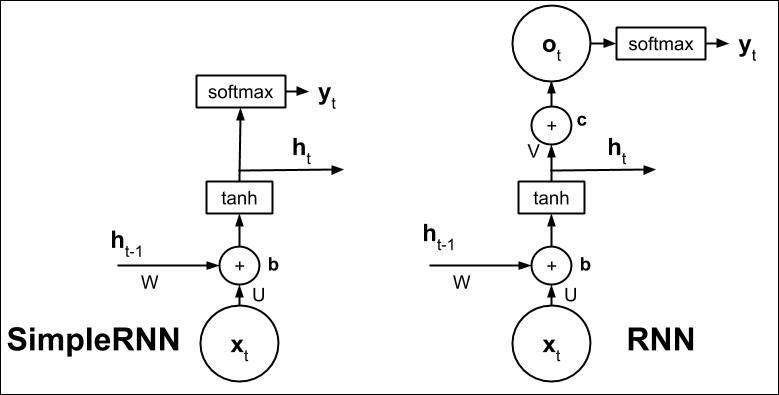Figure 1.5.2: Diagram of SimpleRNN and RNN

RNNs might be initially harder to understand when compared to MLPs or CNNs. In MLPs, the perceptron is the fundamental unit. Once the concept of the perceptron is understood, MLPs are just a network of perceptrons. In CNNs, the kernel is a patch or window that slides through the feature map to generate another feature map. In RNNs, the most important is the concept of self-loop. There is in fact just one cell.

The illusion of multiple cells appears because a cell exists per timestep but in fact, it is just the same cell reused repeatedly unless the network is unrolled. The underlying neural networks of RNNs are shared across cells.

The summary in Listing 1.5.2 indicates that using a `SimpleRNN` requires a fewer number of parameters. Figure 1.5.3 shows the graphical description of the RNN MNIST digit classifier. The model is very concise. Table 1.5.1 shows that the `SimpleRNN` has the lowest accuracy among the networks presented.

Listing 1.5.2, RNN MNIST digit classifier summary:

```_________________________________________________________________
Layer (type)                 Output Shape              Param #
=================================================================
simple_rnn_1 (SimpleRNN)     (None, 256)               72960
_________________________________________________________________
dense_1 (Dense)              (None, 10)                2570
_________________________________________________________________
activation_1 (Activation)    (None, 10)                0
=================================================================
Total params: 75,530
Trainable params: 75,530
Non-trainable params: 0
```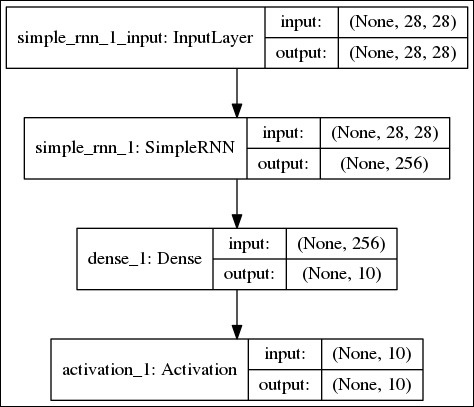Figure 1.5.3: The RNN MNIST digit classifier graphical description

Layers

Optimizer

Regularizer

Train Accuracy, %

Test Accuracy, %

256

SGD

Dropout(0.2)

97.26

98.00

256

RMSprop

Dropout(0.2)

96.72

97.60

256

Dropout(0.2)

96.79

97.40

512

SGD

Dropout(0.2)

97.88

98.30

Table 1.5.1: The different SimpleRNN network configurations and performance measures

In many deep neural networks, other members of the RNN family are more commonly used. For example, Long Short-Term Memory (LSTM) networks have been used in both machine translation and question answering problems. LSTM networks address the problem of long-term dependency or remembering relevant past information to the present output.

Unlike RNNs or SimpleRNN, the internal structure of the LSTM cell is more complex. Figure 1.5.4 shows a diagram of LSTM in the context of MNIST digit classification. LSTM uses not only the present input and past outputs or hidden states; it introduces a cell state, st, that carries information from one cell to the other. Information flow between cell states is controlled by three gates, ft, it and qt. The three gates have the effect of determining which information should be retained or replaced and the amount of information in the past and current input that should contribute to the current cell state or output. We will not discuss the details of the internal structure of the LSTM cell in this book. However, an intuitive guide to LSTM can be found at: http://colah.github.io/posts/2015-08-Understanding-LSTMs.

The `LSTM()` layer can be used as a drop-in replacement to `SimpleRNN()`. If LSTM is overkill for the task at hand, a simpler version called Gated Recurrent Unit (GRU) can be used. GRU simplifies LSTM by combining the cell state and hidden state together. GRU also reduces the number of gates by one. The `GRU()` function can also be used as a drop-in replacement for `SimpleRNN()`.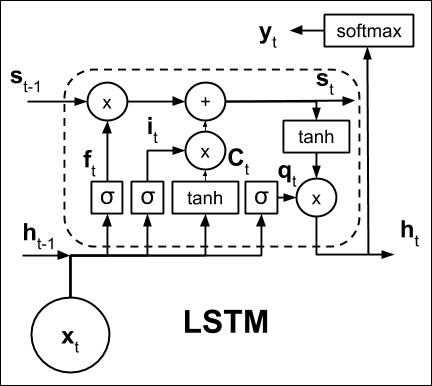Figure 1.5.4: Diagram of LSTM. The parameters are not shown for clarity

There are many other ways to configure RNNs. One way is making an RNN model that is bidirectional. By default, RNNs are unidirectional in the sense that the current output is only influenced by the past states and the current input. In bidirectional RNNs, future states can also influence the present state and the past states by allowing information to flow backward. Past outputs are updated as needed depending on the new information received. RNNs can be made bidirectional by calling a wrapper function. For example, the implementation of bidirectional LSTM is `Bidirectional(LSTM())`.

For all types of RNNs, increasing the units will also increase the capacity. However, another way of increasing the capacity is by stacking the RNN layers. You should note though that as a general rule of thumb, the capacity of the model should only be increased if needed. Excess capacity may contribute to overfitting, and as a result, both longer training time and slower performance during prediction.

## Conclusion

This chapter provided an overview of the three deep learning models – MLPs, RNNs, CNNs – and also introduced Keras, a library for the rapid development, training and testing those deep learning models. The sequential API of Keras was also discussed. In the next chapter, the Functional API will be presented, which will enable us to build more complex models specifically for advanced deep neural networks.

This chapter also reviewed the important concepts of deep learning such as optimization, regularization, and loss function. For ease of understanding, these concepts were presented in the context of the MNIST digit classification. Different solutions to the MNIST digit classification using artificial neural networks, specifically MLPs, CNNs, and RNNs, which are important building blocks of deep neural networks, were also discussed together with their performance measures.

With the understanding of deep learning concepts, and how Keras can be used as a tool with them, we are now equipped to analyze advanced deep learning models. After discussing Functional API in the next chapter, we'll move onto the implementation of popular deep learning models. Subsequent chapters will discuss advanced topics such as autoencoders, GANs, VAEs, and reinforcement learning. The accompanying Keras code implementations will play an important role in understanding these topics.

## References

1. LeCun, Yann, Corinna Cortes, and C. J. Burges. MNIST handwritten digit database. AT&T Labs [Online]. Available: http://yann. lecun. com/exdb/mnist 2 (2010).